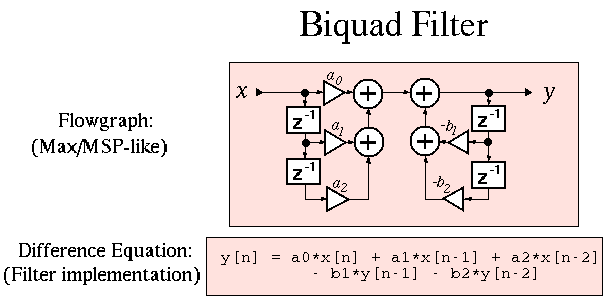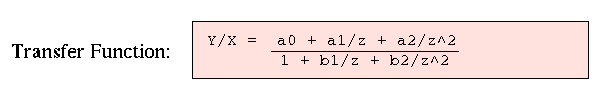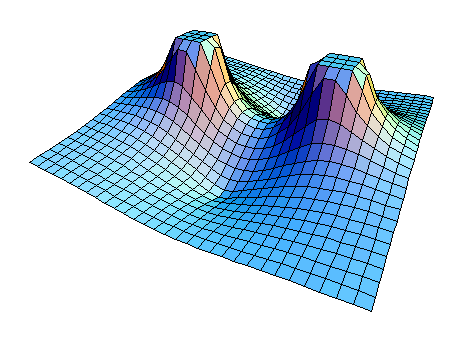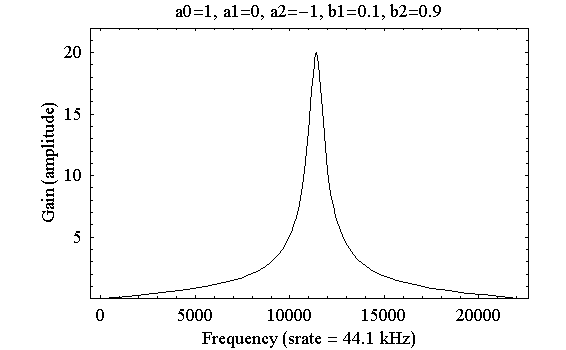# Digital Music Programming II: Biquad filter

A biquad filter is a linear filter, defined by the following flowgraph or difference equation:The name biquad is an abbreviation of the word bi-quadratic because the transfer-function (which can be derived from the difference equation or flowgraph) contains two quadratic functions:Here is an example set of values for the filter coefficients:

```   a0 =  1
a1 =  0
a2 = -1
b1 =  0.1
b2 =  0.9
```

These values will generate the following z-plane magnitude plot:Examing the unit circle in the above z-plane figure, you can determine the spectrum of the filter:Here is a command-line program called biquad.cpp which implements a biquad filter. Save it to your computer and compile it. You can download it directly onto mambo by typing the following command on mambo in the terminal:

```   wget http://peabody.sapp.org/class/dmp2/lab/biquad/biquad.cpp
```
If you need to review how to compile programs, refer to Lab 1.

 ```1 2 3 4 5 6 7 8 9 10 11 12 13 14 15 16 17 18 19 20 21 22 23 24 25 26 27 28 29 30 31 32 33 34 35 36 37 38 39 40 41 42 43 44 ``` ```#include "soundfile.h" int main(int argc, char** argv) { Options options; options.define("a0=d:1", "first feedforward term"); options.define("a1=d:0", "second feedforward term"); options.define("a2=d:-1", "third feedforward term"); options.define("b1=d:0.1", "first feedback term"); options.define("b2=d:0.9", "second feedback term"); options.process(argc, argv); double a0 = options.getDouble("a0"); double a1 = options.getDouble("a1"); double a2 = options.getDouble("a2"); double b1 = options.getDouble("b1"); double b2 = options.getDouble("b2"); SoundFileRead insound(options.getArg(1)); int sampleCount = insound.getSamples(); SoundHeader header = insound; header.setChannels(1); // write only mono files SoundFileWrite outsound(options.getArg(2), header); double currin = 0.0; // x[n] double lastin = 0.0; // x[n-1] double lastin2 = 0.0; // x[n-2] double currout = 0.0; // y[n] double lastout = 0.0; // y[n-1] double lastout2 = 0.0; // y[n-2] // the for-loop implements the difference equation for a biquad filter: // y[n] = a0*x[n] + a1*x[n-1] + a2*x[n-2] - b1*y[n-1] - b2*y[n-2] for (int i=0; i

# Exercises

• Try different values for the filter coeffients. Use whitenoise as an input to the filter program. You can make whitenoise soundfiles on mambo by typing:
```   whitenoise -d 5.0 noise.wav
```
This example would create a five second whitenoise soundfile.

You can set the filter coefficients like this:

```   biquad input.wav output.wav --a0 1 --a1 0 --a2 -1 --b1 0.1 --b2 0.9
or
biquad --a0 1 --a1 0 --a2 -1 --b1 0.1 --b2 0.9 input.wav output.wav
```
```

```